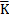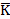NEWS

28

2022

-

01

# Keywords:

rotary,hearth,furnace

# Source: internal company

As the main raw material for direct reduction of rotary hearth furnace, the cold consolidated carbon briqued coal needs to go through transportation, distribution, reduction, discharge and other processes in the production process of rotary hearth furnace, so its performance in all aspects affects the smooth production and efficiency of rotary hearth furnace. In this paper, syrup was used as binder, vanadium titanium magnetite and pulverized coal were used as raw materials to study the influence of different binder ratio, forming pressure and water content on the cold consolidation performance of carbon pellet.

1. The cold consolidation performance of carbon pellet test

1.1 Test raw materials

The ore powder used in the test is vanadium titanomagnetite concentrate, the fixed carbon content of the coal is 82.04%, the volatile matter is 6.58%, and the ash content is 9.88%. The particle size distribution and bulk density of slag and coal powder are shown in Table 1.

Table 1 ：Particle size composition and bulk density of coal powder and ore powder.

 raw material Granular composition % Bulk density (g/ cm-3) +0.4mm 0.15-0.4mm 0.125-0.15mm 0.098-0.125mm 0.074-0.098mm -0.074mm Mineral powder 0.65 20.85 52.80 22.10 2.30 1.30 2.78 coal 21.65 23.25 15.20 17.85 12.70 9.90 0.97

1.2 Research methods

The test process includes several links  such as raw material drying, batching, mixing, pelletizing, drying, and testing. The drying temperature of raw materials was selected as 200 °C, and the drying time was selected as 120 min. The ratio (mass ratio) of ore powder and coal powder is: m (mineral powder): m (coal powder) = 5:1. After the raw materials are evenly mixed, add a certain proportion of binder and distilled water according to the orthogonal table, and stir evenly. The uniformly mixed raw materials are formed by cold consolidation with a roller briquetting machine, and the molding pressure is set according to the orthogonal table. The dried pellets were measured for pellet strength.

2. The cold consolidation performance of carbon pellet results and analysis

2.1 Experimental design and results

After cold consolidation molding, carbon pellets were placed in a drying oven for drying. The drying condition was set at 300 ℃ and the drying time was set at 30 min. After drying, 5 pellets were taken to measure their compressive strength, and then the average value was taken as the compressive strength of pellets. According to the principle of orthogonal test, factor level design is shown in Table 2, and test results are shown in Table 3.

Table 2  Orthogonal test factor levels

 factor level Binder content (factor A)/% Briquette pressure (factor B)/MPa Water addition (factor C)/% 1 4 10 1 2 5 12 2 3 6 15 3 4 7 18 4

Table 3 Experimental scheme and results

 Test no. Binder content (factor A))/% Briquette pressure (factor B)/MPa Water addition (factor C)/% Compressive strength of dry briquettes (P)/N 1 4 10 3 326 2 5 15 1 1631 3 6 12 2 1902 4 7 18 4 606 5 4 18 2 732 6 5 12 4 418 7 6 15 3 1776 8 7 10 1 838 9 4 12 1 473 10 5 18 3 2011 11 6 10 4 437 12 7 15 2 1082 13 4 15 4 417 14 5 10 2 1218 15 6 18 1 1876 16 7 12 3 737

The test results are analyzed in Table 3. SA1 represents the sum of the corresponding test results when factor A takes the first water level, SA2 represents the sum of the corresponding test results when factor A takes the second level, SA3 represents the sum of the corresponding test results when factor A takes the third level, SA4 represents the sum of the corresponding test results at the fourth level of factor A, namely:

SA1 = P1 + P5 + P9 + P13 = 326 + 732 + 473 + 417 = 1948               (1)

SA2 = P2 + P6 + P10 + P14 = 1631 + 418 +2011 + 1218 = 5278           (2)

SA3 = P3 + P7 + P11 + P15 = 1902 + 1776 + 437 + 1876 = 5991         (3)

SA4 = P4 + P8 + P12 + P16 = 606 + 838 + 1082 +737 = 3263          (4)

Divide SA1 , S A2 , S A3 , and S A4 by 4 to get:

KA1 = SA1 /4 = 487                 (5)

KA2 = SA2 /4 = 1320               (6)

KA3 = SA3 /4 = 1498               (7)

KA4 = SA4 /4 = 816                 (8)

KA in equations (5) to (8) represents the spherical compressive strength when the molding pressure and the amount of water added are in the comprehensive average sense, and the amount of binder added is 4%, 5%, 6%, and 7%, respectively . The same method is used to calculate factor B and factor C, and the calculation results are shown in Table 4:

Table 4 Orthogonal analysis of test results

 project factor A factor B factor C S1 1948 2819 4818 S2 5278 3530 4934 S3 5991 4906 4850 S4 3263 5225 1878 K1 487 705 1205 K2 1320 883 1234 K3 1498 1227 1213 K4 816 1306 470 R 1011 601 764

In the orthogonal test, if a certain level factor has a major impact on the results, the quantitative relationship should be shown as a large difference between the comprehensive average K of the indicators under each level of the factor, on the contrary, if the difference between each K is small, it means that the factor is not the main factor. According to the K value in the table, the range of factors A, B and C are calculated as:

RA =1 498 -487 =1 011                               (9)

RB =1 306 -705 =601                                (10)

RC =1 234 -470 =764                                (11)

From Equations (9) to (11), it can be seen that among the three factors A, B and C, the main factor affecting the compressive strength of pellets is the content of binder A, followed by the amount of water added by factor C, and the forming pressure of factor B has relatively little influence on the compressive strength of pellets. Therefore, in order to obtain pellets with high compressive strength, the first step is to choose the appropriate binder ratio.

2.2 The influence of various factors on the test results

In order to describe the influence of various factors on the compressive strength of pellets after forming more clearly, the diagram is made of each factor and the comprehensive average compressive strength value under this factor, as shown in the figureFigure 1 Effect of binder addition, molding pressure and moisture addition on the compressive strength of pellets

It can be seen from Figure 1 that the influences of various factors on the compressive strength of cold-consolidated pellets are different. The compressive strength first increases and then decreases with the increase of the amount of binder added; with the increase of molding pressure, the change trend is always increasing, but the increase is smaller and smaller; when the amount of water added is below 3%, the compressive strength of the pellets has little effect, and the strength of the pellets drops sharply when the amount of water continues to increase.

Based on the analysis of Table 2 and Figure 1, it can be determined that the optimal level combination is A3 B4 C2, that is, the compressive strength of the cold-consolidated pellets when the binder content is 6%, the molding pressure is 18 MPa, and the water content is 2%. Highest, this combination was not included in the 16 trials performed. Therefore, the A3 B4 C2 horizontal combination test was continued, and the average compressive strength of the pellets obtained in the test after drying was 2723 N. This result is higher than the maximum value in the experiment and, therefore, is the optimal combination under the experimental conditions.

## 3. The cold consolidation performance of carbon pellet conclusion

3.1 The effects of three factors, including the amount of binder, the molding pressure and the amount of water added, on the compressive strength of the cold-consolidated pellets were analyzed by orthogonal tests. The compressive strength has the greatest influence, followed by the amount of water added and the molding pressure.

3.2 The influence of the binder on the compressive strength of the pellets after molding is to increase first and then decrease. When 6% syrup is added, the relationship between the molding pressure and the compressive strength of the pellets after molding is that with the increase of the molding pressure, the The compressive strength of the pellets increases continuously; when the amount of water added is not large, it has little effect on the strength of the pellets. When the content exceeds 3%, the strength of the pellets decreases sharply with the increase of the water content.

3.3 The optimal combination A3B4C2 was obtained by the method of orthogonal test, that is, the binder content was 6%, the molding pressure was 18MPa, and the water content was 2%. Under this process condition, the average compressive strength of pellets can reach 2723 N.

Related news

Tel： +86-379-65195189      Fax：+86-379-65182189

Technology Service：+86-13838843223

Add：Unit 1-501/502, Area B, Luoyang National University Science Park

A high-tech enterprise integrating technical consulting, product development and manufacturing, technical services and project operation